﻿ 多级轴流压气机二维性能预测方法Download PDF文章快速检索 高级检索

2D performance prediction approach for multi-stage axial compressors
YANG Xiaofei, JIANG Yongsong, PAN Ruochi, MENG Dejun
AECC Shenyang Engine Research Institute, Shenyang 110015, China
Abstract: This paper conducts a study on the empirical and semi-empirical models for performance prediction of multi-stage axial compressors based on a streamline curvature code. By verifying and integrating the models in literature, a matching loss calculation module is set up, and the endwall loss model is improved. An approach for revising the S2 forward problem is developed with S1 stream surface calculation, and the contradiction between the angle of incidence and deviation angle models based on the data from traditional cascade tests and state-of-the-art airfoils is solved. Then, a S2 analysis program with high solution accuracy is developed. Three experimental compressors with different work coefficients are used to validate the approach. An overall comparison demonstrates that the improved program can have high accuracy and stability, and is applicable for performance analysis of multi-stage axial compressors.
Keywords: multi-stage compressor    performance prediction    loss    incidence angle    deviation angle

1 流线曲率程序

 $\frac{1}{\rho } \cdot \frac{{\partial p}}{{\partial r}} = \frac{{V_\theta ^2}}{r} + \frac{{W_m^2}}{{{r_m}}}\cos \varphi - {W_m}\frac{{{\rm{d}}{W_m}}}{{{\rm{d}}m}}\sin \varphi$ （1）

2 经验模型 2.1 最小损失攻角、落后角模型

 $\begin{array}{l} {i^ * } = {i_{{\rm{m}}1}} = {K_i}{\left( {{i_0}} \right)_{10}} - n{\theta ^\prime }\left( {3 - 4\bar a} \right) + {i_{Ma}}\\ \;\;\;\;\;Ma \le 1.0 \end{array}$ （2）
 ${i^ * } = {i_{{\rm{m}}2}}\;\;\;\;Ma \ge 1.2$ （3）
 ${i^ * } = {i_{{\rm{m}}2}} + \left( {{i_{{\rm{m}}1}} - {i_{{\rm{m}}2}}} \right)f\left( {Ma} \right)\;\;\;\;1.0 < Ma < 1.2$ （4）

 $\delta = \delta _{2{\rm{D}}}^ * + \left( {i - {i^ * }} \right)\frac{{{\rm{d}}{\delta _{2{\rm{D}}}}}}{{{\rm{d}}i}} + {\delta _{\rm{v}}} + {\delta _{3{\rm{D}}}}$ （5）
 $\delta _{2{\rm{D}}}^ * = {K_\delta }{\left( {{\delta _0}} \right)_{10}} + \frac{{{\theta ^\prime }}}{{{\sigma ^b}}}\left[ {{m_{1.0}} + 0.5\left( {\bar a - 0.5} \right)} \right]$ （6）
 ${\delta _{\rm{v}}} = 10\left( {1 - \frac{{{V_{m2}}}}{{{V_{m1}}}}} \right)$ （7）

2.2 设计点损失模型

 ${\omega _{\rm{t}}} = {\omega _{\rm{p}}} + {\omega _{\rm{s}}} + {\omega _{{\rm{sec}}}} + {\omega _{\rm{e}}}$ （8）

2.2.1 叶型损失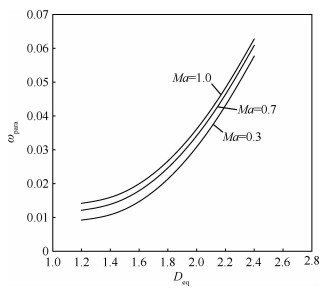图 1 叶型损失参数关系曲线 Fig. 1 Correlation curves for profile loss parameter
 $\begin{array}{l} {D_{{\rm{eq}}}} = \left\{ {1 - \frac{{{V_2}}}{{{V_1}}} + \left[ {0.1 + \bar t\left( {10.116 - 34.15\bar t} \right)} \right] \times } \right.\\ \;\;\;\;\;\;\;\left. {\frac{{{V_{\theta 1}} - {V_{\theta 2}}}}{{{V_1}\sigma }}} \right\}\frac{{{V_1}}}{{{V_2}}} + 1.0 \end{array}$ （9）
 ${\omega _{{\rm{para}}}} = \frac{{{\omega _{\rm{p}}}\cos {\alpha _2}}}{{2\sigma }} \cdot \frac{{V_1^2}}{{V_2^2}}$ （10）

2.2.2 激波损失

Miller模型的关键在于如何计算激波前吸力面马赫数，文献中大都采用普朗特-迈耶函数计算，但这不可避免地会遇到如何求解吸力面气流转角的问题。根据计算校核，本文中激波前吸力面马赫数MaS的计算表达式为

 $\begin{array}{l} M{a_{\rm{S}}} = 1.095 + 0.03395\frac{{0.625}}{\sigma }\Delta \beta + \\ \;\;\;\;\;\;\;1.086{\left( {M{a_1} - 1.0} \right)^{1.372}} \end{array}$ （11）

2.2.3 二次流损失

 ${\omega _{{\rm{sec}}}} = {\omega _{\rm{t}}}\left( {\frac{{{f_{\rm{s}}}}}{{1 + {f_{\rm{s}}}}}} \right)$ （12）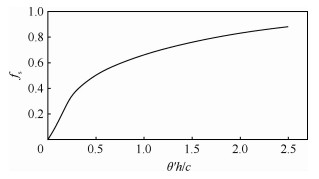图 2 二次流损失因子曲线 Fig. 2 Curve for secondary loss factor
2.2.4 端壁损失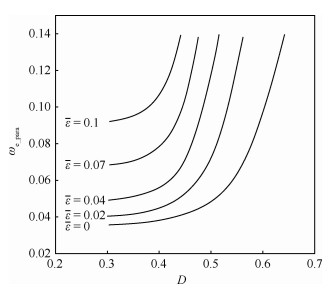图 3 端壁损失参数随扩散因子和间隙的变化 Fig. 3 Variation of endwall loss parameter with diffusion factor and clearance
 $D = 1 - \frac{{{V_2}}}{{{V_1}}} + \frac{{{r_1}{V_{\theta 1}} - {r_2}{V_{\theta 2}}}}{{\left( {{r_2} + {r_1}} \right){V_1}}}$ （13）
 ${\omega _{{\rm{e\_para}}}} = {\omega _{\rm{e}}}\frac{h}{c} \cdot \frac{{V_1^2}}{{V_2^2}}$ （14）

 ${K_j} = {\omega _{\rm{e}}}/{\omega _{\rm{p}}}\;\;\;j = {\rm{H}},{\rm{T}}$ （15）

 ${\omega _{\rm{e}}} = {\omega _{\rm{p}}}{K_{\rm{H}}}{\left( {1 - 2.0\bar h} \right)^{\rm{M}}}\;\;\;\;\bar h < 0.3$ （16）
 ${\omega _{\rm{e}}} = {\omega _{\rm{p}}}{K_{\rm{T}}}{\left( {2.0\bar h - 1} \right)^M}\;\;\;\;\bar h \ge 0.7$ （17）

2.3 非设计点损失模型

 $\omega = {\omega _{\rm{t}}}\left[ {5 - 4\left( {\xi + 2} \right)} \right]\;\;\;\;\xi < - 2$ （18）
 $\omega = {\omega _{\rm{t}}}\left( {1 + {\xi ^2}} \right)\;\;\;\; - 2 \le \xi \le 1$ （19）
 $\omega = 2{\omega _{\rm{t}}}\xi \;\;\;\xi > 1$ （20）

 $\cos {\beta _{{\rm{cr}}}} = \frac{o}{s}{\left( {\frac{{k + 1}}{2}} \right)^{\frac{1}{{1 - k}}}}{\left( {1 - \frac{{k - 1}}{{k + 1}}\lambda _1^2} \right)^{\frac{1}{{1 - k}}}}\frac{1}{{{\lambda _1}}}$ （21）

 ${\rm{WIDTH}} \ge {\beta _{{\rm{cr}}}} - \beta _1^ *$ （22）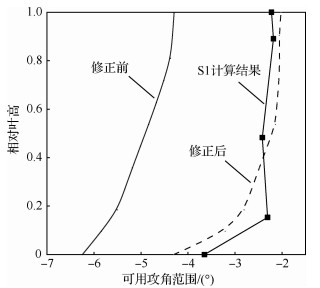图 4 可用攻角范围对比 Fig. 4 Comparison of available angle of incidence range
2.4 径向掺混

3 S1流面计算的应用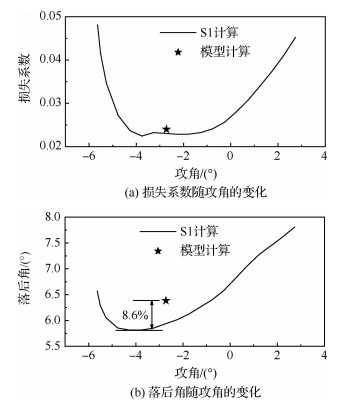图 5 NACA-65叶型计算结果 Fig. 5 Calculation results of NACA-65 blade profile图 6 定制叶型计算结果 Fig. 6 Calculation results of tailored blade profile

 $i_{\rm{M}}^ * = i_{{{\rm{S}}_1}}^ *$ （23）
 $\delta _{\rm{M}}^ * = \delta _{{{\rm{S}}_1}}^ * + {C_1}$ （24）
 $\delta _j^ * = \delta _{{{\rm{S}}_1}}^ * + {C_2}\;\;\;\;j = {\rm{H}},{\rm{T}}$ （25）

iM*=iS1*通过调整攻角模型的叶型修正系数实现，其余流面的叶型修正系数与叶中流面相同，从而可以确定各个流面的最小损失攻角。对于落后角，除根、中、尖3个流面外，其余流面的落后角保证展向分布光顺。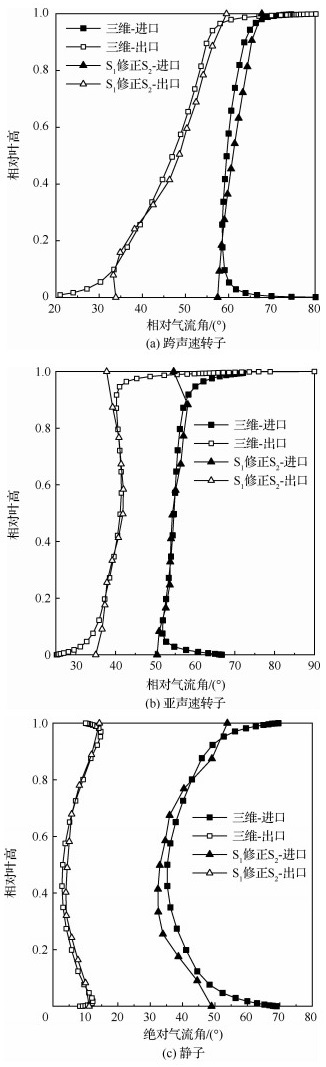图 7 跨声速转子、亚声速转子和静子的气流角对比 Fig. 7 Comparison of airflow angles of transonic rotor, subsonic rotor and stator

4 失速模型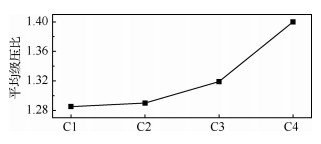图 8 4台压气机的平均级压比 Fig. 8 Average stage pressure ratio of four compressors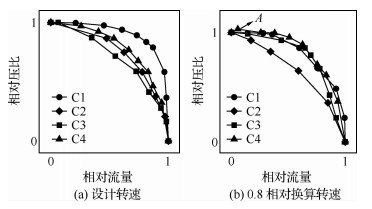图 9 归一化试验特性 Fig. 9 Normalized test characteristics

5 校验计算

 $H = \frac{{{L_{\rm{u}}}}}{{U_{\rm{T}}^2}}$ （26）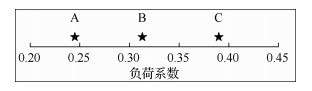图 10 压气机负荷系数 Fig. 10 Work coefficient of compressors

5.1 A压气机

A压气机为面向燃气轮机的低压压气机，压气机前两级转子存在局部超声速区，一级转子叶尖进口相对马赫数为1.16。压气机转、静子叶片均采用修正圆弧中线、NACA-65叶厚分布。为适应不同叶片的进口马赫数及流通能力，中弧线采用了不同程度的加弯或减弯。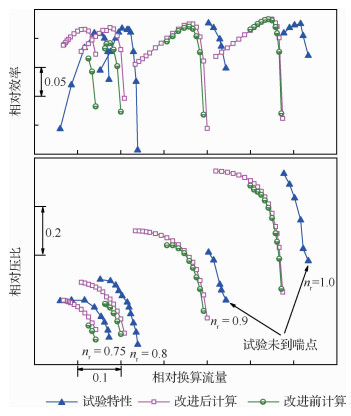图 11 A压气机特性 Fig. 11 Overall performance of Compressor A

5.2 B压气机

B压气机为E3十级高压压气机第2轮修改后的压气机。E3十级高压压气机在1.0换算转速的设计流量为54.4 kg/s，总压比为25.0，效率为0.847，一级转子叶尖进口相对马赫数为1.353。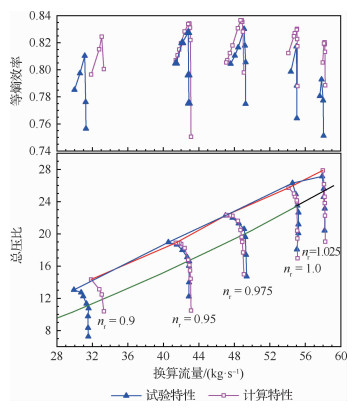图 12 B压气机(E3十级压气机)特性 Fig. 12 Overall performance of Compressor B (E3 ten-stage compressor)

0.9相对转速计算与试验偏差较大，这除了计算给定的间隙较小外，还与进口导流叶片的性能计算有关，转速降低后进口导流叶片关角度值很大，出口气流角及总压恢复系数计算精度降低。

5.3 C压气机

C压气机为高负荷多级压气机，前3级转子叶片存在超声速区域，一级转子叶尖进口相对马赫数为1.22。各排叶片均采用多圆弧叶型。在特性计算前，首先计算了各排叶片根、中、尖3个流面的叶型攻角特性，对最小损失攻角和落后角进行了修正，随后开展了全转速特性预估。计算特性如图 13所示，计算与试验吻合良好。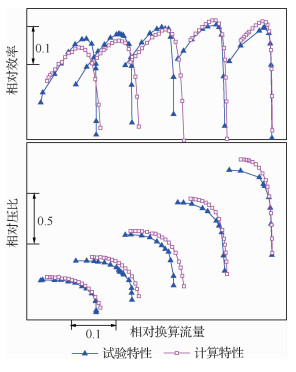图 13 C压气机特性 Fig. 13 Overall performance of Compressor C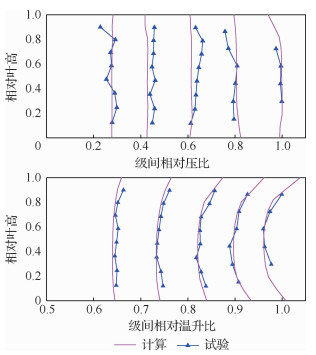图 14 近堵塞点的压力和温度对比 Fig. 14 Comparison of pressure and temperature near choked operating point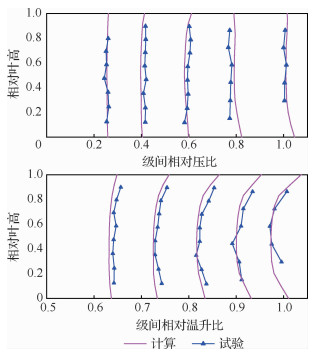图 15 近设计点的压力和温度对比 Fig. 15 Comparison of pressure and temperature near design operating point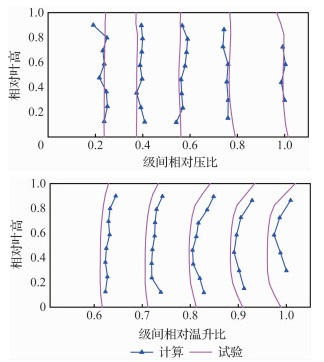图 16 近失速点的压力和温度对比 Fig. 16 Comparison of pressure and temperature near stall operating point
6 结论

1) 除低负荷压气机的计算流量偏低外，采用本研究发展的方法计算的压气机性能与试验结果吻合良好，展现出相对较高的计算精度。

2) 本文发展的方法合理，较大幅度提高了程序的计算稳定性，可用于多级轴流压气机性能分析，为压气机多程序校核提供了新手段。

http://dx.doi.org/10.7527/S1000-6893.2019.23515

0

#### 文章信息

YANG Xiaofei, JIANG Yongsong, PAN Ruochi, MENG Dejun

2D performance prediction approach for multi-stage axial compressors

Acta Aeronautica et Astronautica Sinica, 2020, 41(3): 123515.
http://dx.doi.org/10.7527/S1000-6893.2019.23515